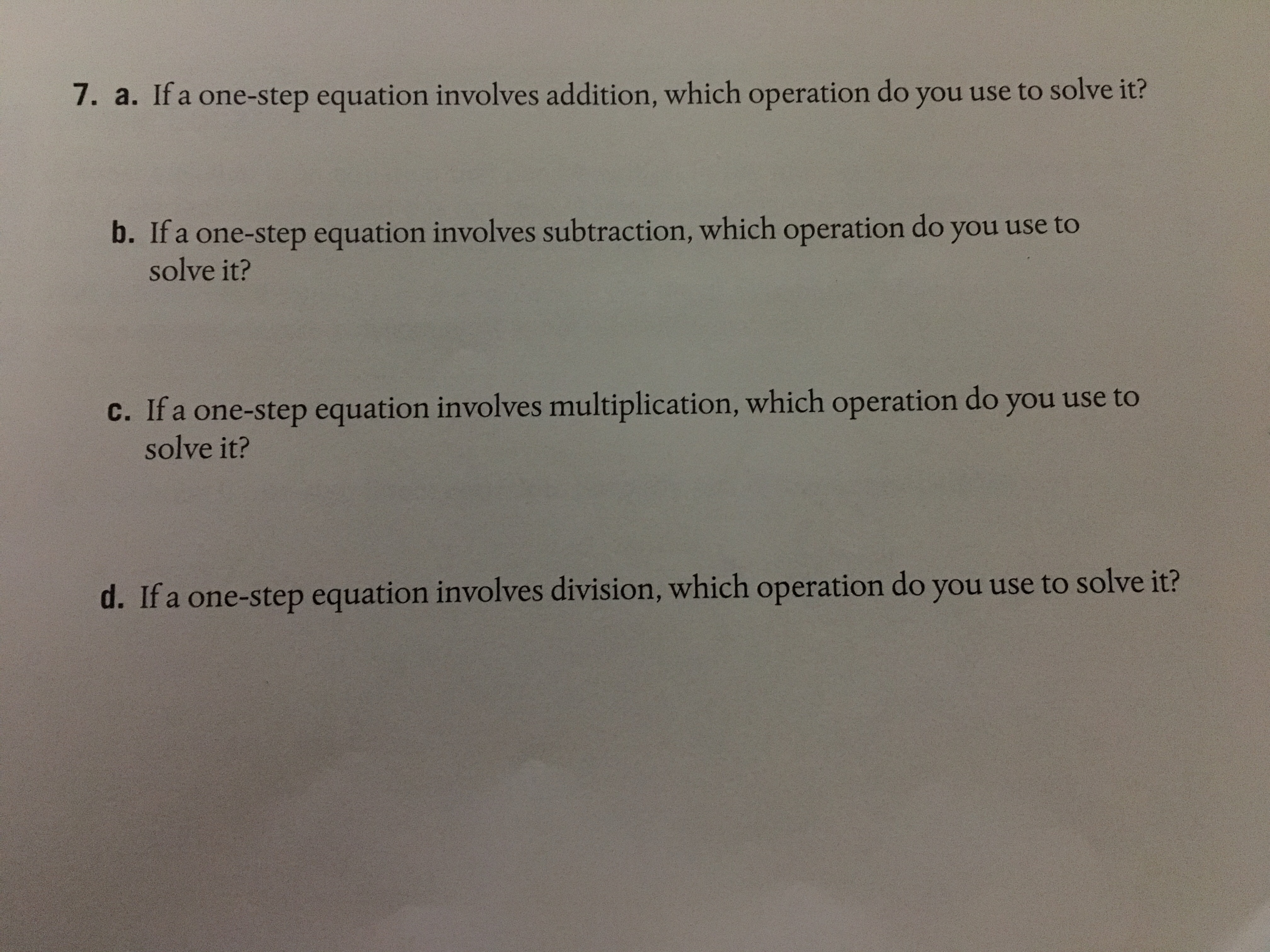# 7. a. If a one-step equation involves addition, which operation do you use to solve it? b. If a one-step equation involves subtraction, which operation do you use to solve it? c. Ifa one-step equation involves multiplication, which operation do you use to solve it? d. If a one-step equation involves division, which operation do you use to solve it?

Questionhelp_outlineImage Transcriptionclose7. a. If a one-step equation involves addition, which operation do you use to solve it? b. If a one-step equation involves subtraction, which operation do you use to solve it? c. Ifa one-step equation involves multiplication, which operation do you use to solve it? d. If a one-step equation involves division, which operation do you use to solve it? fullscreen

### Want to see this answer and more?

Experts are waiting 24/7 to provide step-by-step solutions in as fast as 30 minutes!*